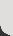﻿ Mental calculation strategies - addition and subtraction - (TLF R10912 v4.0.0)

This collection of 21 digital curriculum resources focuses on assisting students to develop efficient and effective mental calculation strategies for performing additions and subtractions of two-, three- and four-digit whole numbers. It is organised in three categories: developing strategies - addition; developing strategies - subtraction; and applying strategies. The ten learning objects in the applying strategies section give students opportunities to practise addition and subtraction strategies by making their own problems or by using a game-like environment.

L103 The number partner

L105 The number partner: go figure

L96 The part-adder: go figure

L114 The difference bar: go figure

L102 The take-away bar: go figure